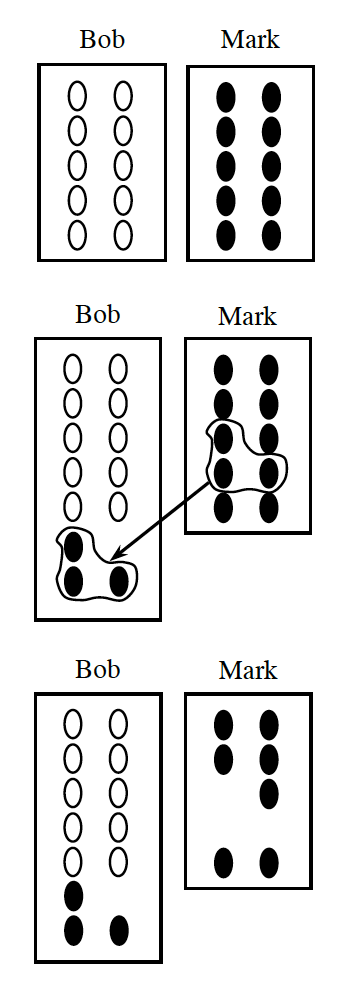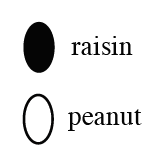### Home > CC1 > Chapter 1 > Lesson 1.1.5 > Problem1-36

1-36.

Bob and Mark decided to try the peanut and raisin investigation at home. Bob started with $10$ peanuts on his tray, and Mark started with $10$ raisins on his tray, as shown in the diagram at right.

1. Mark gave $3$ raisins to Bob, as shown in the diagram at right. How does the number of peanuts and raisins on Bob’s tray compare to the number of peanuts and raisins on Mark’s tray?

It may be helpful to use real peanuts/raisins (or substitutes) and re-enact this situation.

Bob has $10$ peanuts and $3$ raisins. Mark has $7$ raisins.

2. Copy the diagram below right. Circle a group of any $3$ peanuts and raisins on Bob’s tray. Be sure to circle some of each for a total of $3$. Then give them to Mark. Does Bob now have more of Mark’s raisins, or does Mark have more of Bob’s peanuts?

First, what would happen if Bob gave Mark $2$ peanuts and $1$ raisin. Next, what would happen if Bob gave Mark $1$ peanut and $2$ raisins.

Bob has the same number of Mark's raisins as Mark has of Bob's peanuts.

3. Now start from the beginning and repeat parts (a) and (b) assuming that $8$ (instead of $3$) snack items are handed from one student to the other. Explain your results.

Repeat the processes from parts (a) and (b), but use $8$ snack items instead of $3$.